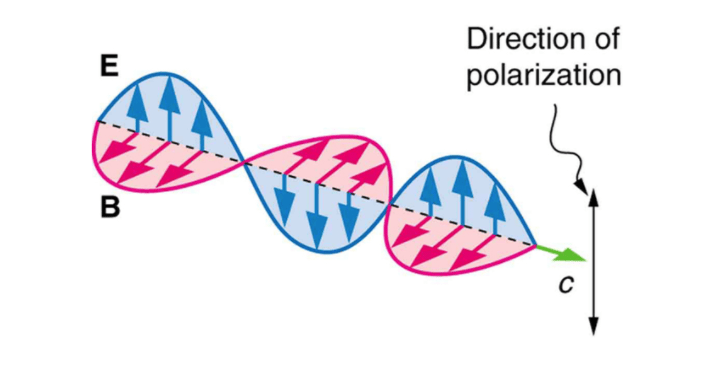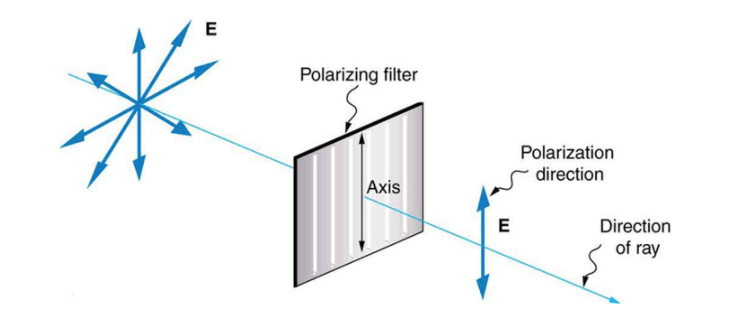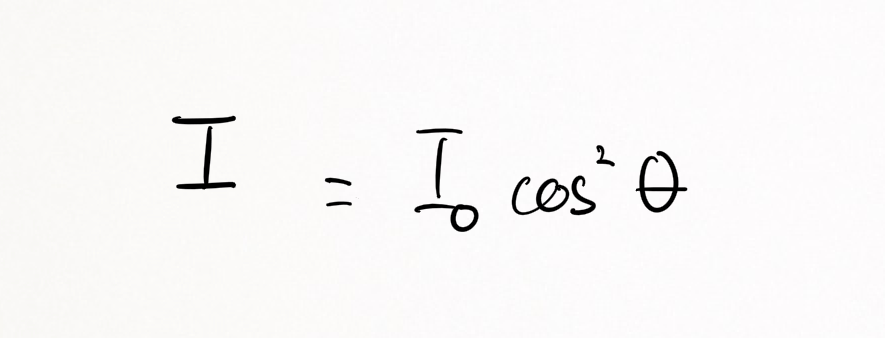Complimentary 1-hour tutoring consultation

MCAT Content / Light And Electromagnetic Radiation / Polarization Of Light Linear And Circular

### Polarization of light: linear and circular

Electromagnetic waves are polarized if their electric field vectors are all in a single plane (linear polarization), or in 2 plans perpendicular to each other, equal in amplitude, but have a phase difference of π/2 (circular polarization).

Light waves from common sources (sunlight, halogen lighting, LED spotlights, and incandescent bulbs) are unpolarized, or polarized randomly.When a polarizing sheet is placed in the path of light, only electric field components of the light parallel to the sheet’s polarizing direction are transmitted by the sheet; components perpendicular to the polarizing direction are absorbed. The light that emerges from a polarizing sheet is polarized parallel to the polarizing direction of the sheet.If the original light is initially unpolarized, the transmitted intensity I is half the original intensity.If the original light is initially polarized, the transmitted intensity depends on the angle (θ )between the polarization direction of the original light and the polarizing direction of the sheet:Polarization can occur in two forms. Linear polarization where the electric field of light is confined to a single plane along the direction of propagation. Or circular polarization, where the electric field of the light consists of two linear components that are perpendicular to each other, equal in amplitude but have a phase difference of π/2. The resulting electric field rotates in a circle around the direction of propagation and, depending on the rotation direction, is called left- or right-hand circularly polarized light.

Key Points

• There is linear polarization (confined to a single plane) and circular polarization (two linear components that are perpendicular to each other.)

• If the original light is initially unpolarized, the transmitted intensity I is half the original intensity. If the original light is initially polarized, the transmitted intensity depends on the angle θ.

Key Terms

unpolarized: electric field vector of EM wave is in all directions

Billing Information